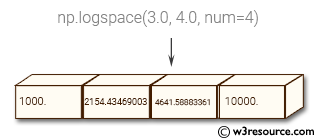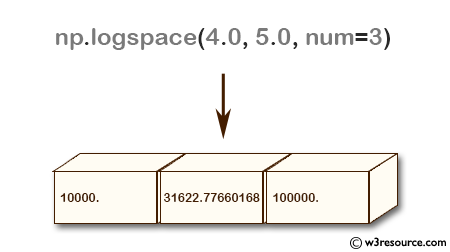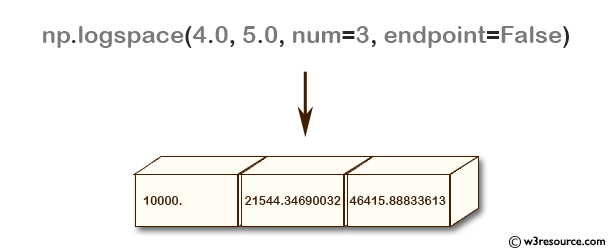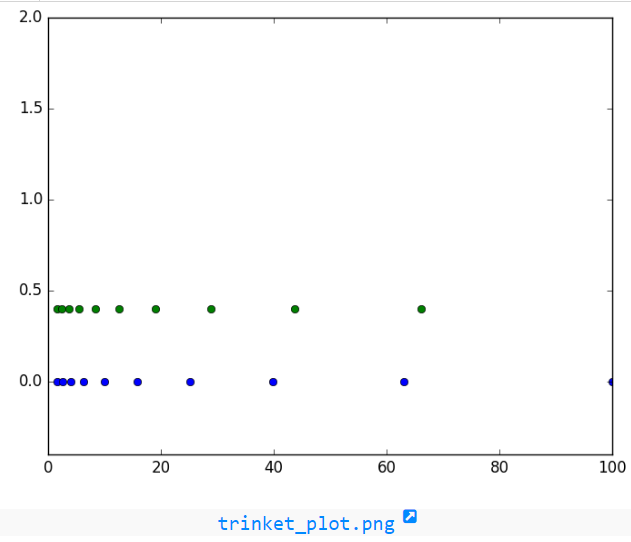# NumPy array creation: logspace() function

## numpy.logspace() function

The logspace() function return numbers spaced evenly on a log scale.
In linear space, the sequence starts at base ** start (base to the power of start) and ends with base ** stop.

Syntax:

`numpy.geomspace(start, stop, num=50, endpoint=True, dtype=None)`Version: 1.15.0

Parameter:

Name Description Required /
Optional
start base ** start is the starting value of the sequence.
stop base ** stop is the final value of the sequence, unless endpoint is False. In that case, num + 1 values are spaced over the interval in log-space, of which all but the last (a sequence of length num) are returned.
num Number of samples to generate. Default is 50. optional
endpoint If True, stop is the last sample. Otherwise, it is not included. Default is True. optional
base The base of the log space. The step size between the elements in ln(samples) / ln(base) (or log_base(samples)) is uniform. Default is 10.0. optional
dtype The type of the output array. If dtype is not given, infer the data type from the other input arguments.

Return value:

samples : ndarray - num samples, equally spaced on a log scale.

Example-1: NumPy.logspace() function

``````>>> import numpy as np
>>> np.logspace(4.0, 5.0, num=3)
array([  10000.        ,   31622.77660168,  100000.        ])
>>> np.logspace(4.0, 5.0, num=3, endpoint=False)
array([ 10000.        ,  21544.34690032,  46415.88833613])
>>> np.logspace(4.0, 5.0, num=3, base=2.0)
array([ 16.      ,  22.627417,  32.      ])
``````

Pictorial Presentation:Example-2: NumPy.logspace() function

``````>>> import numpy as np
>>> import matplotlib.pyplot as plt
>>> A=10
>>> a1=np.logspace(0.2, 2, A, endpoint=True)
>>> a2=np.logspace(0.2, 2, A, endpoint=False)
>>> b=np.zeros(A)
>>> plt.plot(a1, b, 'o')
[]
>>> plt.plot(a2, b + 0.4, 'o')
[]
>>> plt.ylim([-0.4, 2])
(-0.4, 2)
>>> plt.show()
``````

numpy.logspace.plot showPython - NumPy Code Editor:

Previous: linspace()
Next: geomspace()

﻿